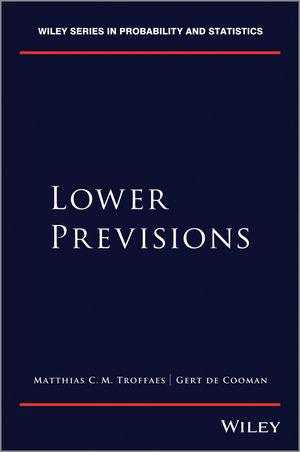## Lower Previsions: The Book

This page gives some further information about the Lower Previsions book, published by myself and Gert de Cooman (Wiley Series in Probability and Statistics, 2014).### Errata

Warmest thanks to Paolo Vicig, Marshall Abrams, Jasper de Bock, Fabio Cozman, Nawapon Nakharutai, and Tathagata Basu for reporting some of these errata.

• p10, line above Definition 1.14: "μ(A)⊆μ(B)" should be "μ(A)≤μ(B)"
• p16, Lemma 1.21(iii): "so the sequence uₙ ∘ f + uₙ ∘ g converges uniformly to f + g" should be "so the sequence uₙ ∘ (f + g) converges uniformly to f + g"
• p29, line 1: "a acceptable" should be "an acceptable"
• p31: The text says that Walley's 2000 notion of desirability captures a weak preference to the zero gamble, whilst his 1991 notion captures strong preference. It must be the other way around: "Our notion of acceptability coincides with Walley's earlier (1991, Appendix F) notion of desirability, also used by Moral (2000) and Couso and Moral (2009, 2011), and aims at capturing a weak preference to the zero gamble. Walley in his later paper (2000, p. 137) and also Moral (2005) use a slightly different notion of acceptability, which is rather aimed at representing a strict preference to the zero gamble."
• p38, middle of page: "Similarly, upr(D)(f) is the *infimum* price"
• p39, proof of Theorem 4.2: The proof of the equality "sup{μ∈ℝ:f-μ∈D}=P̲(f)" is incomplete. To correct the proof, replace "P̲(f)<μ⇒P̲(f-μ)<0⇒f-μ∉D" by "f-μ∉D⇒(P̲(f-μ)≤0 and f-μ≱0)⇒P̲(f)≤μ".
• p42: The inequality just before Definition 4.4 must be reversed: "P̲(f)≤Q̲(f)".
• p44, line 2: "j∈{1, ..., n}" should be "i∈{1, ..., n}"
• p45, paragraph before 4.2.4: "transacttion" should be "transaction"
• p47, Definition 4.10(D): "bounded gambles f₀, ..., fₘ" should be "bounded gambles f₀, ..., fₙ"
• p48, 2nd line of (C)=>(D): "bounded gambles f₀, ..., fₘ" should be "bounded gambles f₀, ..., fₙ"
• p51, section 4.3.4, first paragraph: "P(-f)=P(f)" should be "P(-f)=-P(f)"
• p58, Corollary 4.17: "all all" should be "all"
• p58, paragraph above Proposition 4.18: "defined *on* a linear subspace"
• p66, paragraph above section 4.5.2: "and therefore of P̲" should be "and therefore P̲"
• p68, equation (4.10c): all indices should be k instead of i
• p105, equation in proof of Proposition 6.5: The "f∧" is not carried through: after the first equality sign we should have "P̲(r(f∧...", and after the second equality sign we should have "P̲(r(f)∧...".
• p107, bottom: "This inequality *is* trivially satisfied ..."
• p110, Proposition 6.12: "Assume that P̲ is non-negative *and monotone* ..."
• p123, line just above equation (7.2): "p̲(A)" should be "p̲(x)"
• p233, fourth last line: "... that the subject is practically *certain* will only ..."
• p283, Theorem 13.53, item (iv) should be instead: "For all non-empty events A there is a gamble f such that -∞<E̲(f|A)<+∞."
• p284-285, proof of Theorem 13.53 (iv) => (i): The last step of the proof (going from (13.28) to the equation on the page 285) is wrong. To fix the proof, choose A to be the union of C₁, ..., Cₚ and assume that E̲(f|A)>-∞. Now you can easily prove that E̲(f|A)=+∞.
• p375, last paragraph: the phrases "all measurable bounded gambles are integrable" and "equivalence of Lebesgue integration and natural extension" should appear between brackets
• p381, proof of Colollary C.4, line 2: the sum should start at k=2 instead of k=1
• p382, top line: "on the set B *of* all bounded gambles"
• p396, fourth entry: gamble -> Gamble

If you think you found any other errors, please contact me.